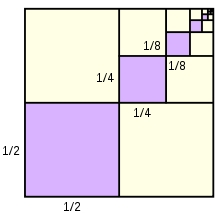# Difference 10741

Twice, the difference between the number 50 and the unknown x reduced by the unknown x is equal to the number 106. What is the unknown number?

x =  -2

### Step-by-step explanation:

2(50-x) - x = 106

2·(50-x) - x = 106

3x = -6

x = -6/3 = -2

x = -2

Our simple equation calculator calculates it.Did you find an error or inaccuracy? Feel free to write us. Thank you!

Tips for related online calculators
Do you have a linear equation or system of equations and looking for its solution? Or do you have a quadratic equation?